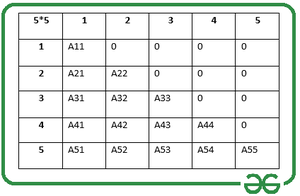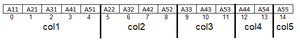Related Articles

# Efficient method to store a Lower Triangular Matrix using Column-major mapping

• Last Updated : 07 Jul, 2021

Given a lower triangular matrix Mat[][], the task is to store the matrix using column-major mapping.

Lower Triangular Matrix: A Lower Triangular Matrix is a square matrix in which the lower triangular part of a matrix consists of non-zero elements and the upper triangular part consists of 0s. The Lower Triangular Matrix for a 2D matrix Mat[][] is mathematically defined as:

• If i < j, set Mat[i][j] = 0.
• If i >= j, set Mat[i][j] > 0.

Illustration:

Below is a 5×5 lower triangular matrix. In general, such matrices can be stored in a 2D array, but when it comes to matrices of large size, it is not a good choice because of its high memory consumption due to the storage of unwanted 0s
Such a matrix can be implemented in an optimized manner.The efficient way to store the lower triangular matrix of size N:

• Count of non-zero elements = 1 + 2 + 3 + … + N = N * (N + 1) /2.
• Count of 0s = N2 – (N * (N + 1) /2 = (N * (N – 1)/2.

Now let see how to represent lower triangular matrices in the program. Notice that storing 0s must be avoided to reduce memory consumption. As calculated, for storing non-zero elements, N*(N + 1)/2 space is needed. Taking the above example, N = 5. Array of size 5 * (5 + 1)/2 = 15 is required to store the non-zero elements.

Now, elements of the 2D matrix can be stored in a 1D array, column by column, as shown below:Array to store Lower Triangular Elements

Apart from storing the elements in an array, a procedure for extracting the element corresponding to the row and column number is also required. Using Column-Major-Maping for storing a lower triangular matrix, the element at index Mat[i][j] can be represented as:

Index of Mat[i][j] matrix in the array A[] = [n*(j-1)-(((j-2)*(j-1))/2)+ (i-j))]

Below is the implementation of the above article:

## C++

 `// C++ program for the above approach``#include ``#include``using` `namespace` `std;` `// Dimensions of the matrix``const` `int` `N = 5;` `// Structure of a memory``// efficient matrix``struct` `Matrix {``    ``int``* A;``    ``int` `size;``};` `// Function to set the``// values in the Matrix``void` `Set(``struct` `Matrix* m, ``int` `i,``         ``int` `j, ``int` `x)``{``    ``if` `(i >= j)``        ``m->A[((m->size)*(j-1)-(((j-2)``              ``*(j-1))/2)+(i-j))] = x;``}` `// Function to store the``// values in the Matrix``int` `Get(``struct` `Matrix m, ``int` `i, ``int` `j)``{``    ``if` `(i >= j)``        ``return` `m.A[((m.size)*(j-1)-(((j-2)``                   ``*(j-1))/2)+(i-j))];``    ``else``        ``return` `0;``}` `// Function to display the``// elements of the matrix``void` `Display(``struct` `Matrix m)``{``    ``// Traverse the matrix``    ``for` `(``int` `i = 1; i <= m.size; i++)``    ``{``        ``for` `(``int` `j = 1; j <= m.size; j++)``        ``{``            ``if` `(i >= j)``                ``cout<< m.A[((m.size)*(j-1)-(((j-2)``                       ``*(j-1))/2)+(i-j))] <<``" "``;``            ``else``                ``cout<<``"0 "``;``        ``}``        ``cout<

## C

 `// C program for the above approach``#include ``#include ` `// Dimensions of the matrix``const` `int` `N = 5;` `// Structure of a memory``// efficient matrix``struct` `Matrix {``    ``int``* A;``    ``int` `size;``};` `// Function to set the``// values in the Matrix``void` `Set(``struct` `Matrix* m, ``int` `i,``         ``int` `j, ``int` `x)``{``    ``if` `(i >= j)``        ``m->A[((m->size)*(j-1)-(((j-2)``              ``*(j-1))/2)+(i-j))] = x;``}` `// Function to store the``// values in the Matrix``int` `Get(``struct` `Matrix m, ``int` `i, ``int` `j)``{``    ``if` `(i >= j)``        ``return` `m.A[((m.size)*(j-1)-(((j-2)``                   ``*(j-1))/2)+(i-j))];``    ``else``        ``return` `0;``}` `// Function to display the``// elements of the matrix``void` `Display(``struct` `Matrix m)``{``    ``// Traverse the matrix``    ``for` `(``int` `i = 1; i <= m.size; i++)``    ``{``        ``for` `(``int` `j = 1; j <= m.size; j++)``        ``{``            ``if` `(i >= j)``                ``printf``(``"%d "``,``                       ``m.A[((m.size)*(j-1)-(((j-2)``                       ``*(j-1))/2)+(i-j))]);``            ``else``                ``printf``(``"0 "``);``        ``}``        ``printf``(``"\n"``);``    ``}``}`  `// Function to generate an efficient matrix``struct` `Matrix createMat(``int` `Mat[N][N])``{``    ``// Declare efficient Matrix``    ``struct` `Matrix mat;``  ` `    ``// Initialize the Matrix``    ``mat.size = N;``    ``mat.A = (``int``*)``malloc``(``        ``mat.size * (mat.size + 1) / 2``        ``* ``sizeof``(``int``));``  ` `    ``// Set the values in matrix``    ``for` `(``int` `i = 1; i <= mat.size; i++) {``  ` `        ``for` `(``int` `j = 1; j <= mat.size; j++) {``  ` `            ``Set(&mat, i, j, Mat[i - 1][j - 1]);``        ``}``    ``}``  ` `    ``// Return the matrix``    ``return` `mat;``}`  `// Driver Code``int` `main()``{``    ``// Given Input``    ``int` `Mat = { { 1, 0, 0, 0, 0 },``                      ``{ 1, 2, 0, 0, 0 },``                      ``{ 1, 2, 3, 0, 0 },``                      ``{ 1, 2, 3, 4, 0 },``                      ``{ 1, 2, 3, 4, 5 } };``    ` `    ``// Function call to create a memory``    ``// efficient matrix``    ``struct` `Matrix mat = createMat(Mat);``  ` `    ``// Function call to``      ``// print the Matrix``    ``Display(mat);` `    ``return` `0;``}`

Output
```1 0 0 0 0
1 2 0 0 0
1 2 3 0 0
1 2 3 4 0
1 2 3 4 5 ```

Time Complexity: O(N2)
Auxiliary Space: O(N2)

Attention reader! Don’t stop learning now. Get hold of all the important DSA concepts with the DSA Self Paced Course at a student-friendly price and become industry ready.  To complete your preparation from learning a language to DS Algo and many more,  please refer Complete Interview Preparation Course.

In case you wish to attend live classes with experts, please refer DSA Live Classes for Working Professionals and Competitive Programming Live for Students.

My Personal Notes arrow_drop_up